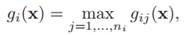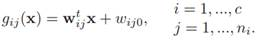# A classifier is said to be a piecewise linear machine if its discriminant functions linear machine have the form Where (a) Indicate how a piecewise linear machine can be viewed in terms of a linear machine for classifying subclasses of patterns. (b) Show that the decision regions of a piecewise linear machine can be nonconvex and even multiply connected. (c) Sketch a plot of gij (x) for a one-dimensional example in which n1 = 2 and n2 = 1 to illustrate your answer to part (b).

Question
1 views

A classifier is said to be a piecewise linear machine if its discriminant functions linear machine have the formWhere(a) Indicate how a piecewise linear machine can be viewed in terms of a linear machine for classifying subclasses of patterns.

(b) Show that the decision regions of a piecewise linear machine can be nonconvex and even multiply connected.

(c) Sketch a plot of gij (x) for a one-dimensional example in which n1 = 2 and n2 = 1 to illustrate your answer to part (b).

### This question hasn't been answered yet.